Back to Contents

# Project 3: Noughts and Crosses

The childhood game of Noughts and Crosses is a combinatorial problem of a large but manageable size. Let us review the rules, and then see if we can build the game tree (that is, a structure describing all possible games), and draw some statistics from it.

A 3-by-3 grid is constructed. Two players (O and X) take turns to place their piece in an empty space. The game is won if or when a player forms three pieces in a row, column, or diagonally. The game is drawn if the board is full and no such pattern has been formed. For example, in this board, player O has won, building a diagonal: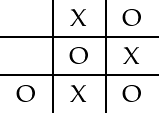Question 1 Games of size 1-by-1 and 2-by-2 are uninteresting. Why?

Question 2 From your own experience, can you write down some rules to help a beginning player win (or avoid losing) a 3-by-3 game of Noughts and Crosses?

### Representing the board

We can assign a number to each position in the board: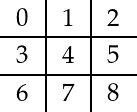The board can be represented as a simple list of strings, one for each position 0..8 in the board. In the empty board, all positions have the string `’_’`.

``emptyboard = ['_', '_', '_', '_', '_', '_', '_', '_', '_']``

You might wonder why we do not use a list of lists of length three to represent rows. This does not in fact simplify the problem, since we need to talk about columns and diagonals just as often. We can print the board out: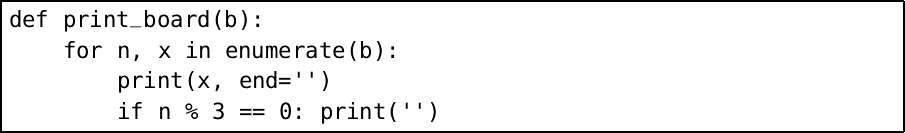We will need some simple functions to determine properties of a board. To test when a board is full (i.e. when the game is drawn) is easy: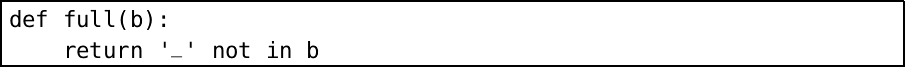Calculating whether a particular player has won is rather harder, since we need to check each horizontal line, vertical line, and diagonal. We can enumerate these lines:

`h1 = [0, 1, 2]` horizontal
`h2 = [3, 4, 5]`
`h3 = [6, 7, 8]`
`v1 = [0, 3, 6]` vertical
`v2 = [1, 4, 7]`
`v3 = [2, 5, 8]`
`d1 = [0, 4, 8]` diagonal
`d2 = [2, 4, 6]`

`lines = [h1, h2, h3, v1, v2, v3, d1, d2]`

Now we can look up each line’s positions in the board, and compare it with what a winning line would look like: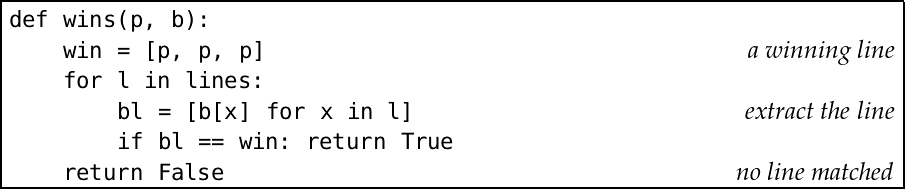Question 3 Write a function `random_move` which plays a given piece in a blank space, chosen at random. Now write a function `random_game` which uses it to play an arbitrary, but valid, game. The game should end when it is won or drawn, even if the board is not yet full. Print the game as it progresses.

### Human players

The human player plays `’O’`. The `human_move` function asks the user to select a board position for the next `’O’`, making sure to check the user’s input is sensible. Then it fills in the space on the board.

``````Python
>>> b = ['_', 'X', '_', '_', '_', '_', '_', '_', '_']
>>> human_move(b)
Position 0..8? six
Not a valid board position
Position 0..8? 9
Board position must be from 0..8
Position 0..8? 5
>>> b
['_', 'X', '_', '_', '_', 'O', '_', '_', '_']``````

Question 4 Write the function `human_move` matching this description. The built-in method `isdigit` on strings may be used to check if a string represents a digit.

Question 5 Extend the function to play either `’X’` or `’O’`. Now write a program allowing two humans to play one another. Print the empty board at the beginning, and the board after each turn. Print which player won, or if it was a draw.

### Computer players

In question 3, we wrote a very simple random computer player. This is a player against which a human would almost always win. As we know from experience, however, even a child can learn easily how to win (or force a draw) in any game of 3x3 Noughts and Crosses, whether they place the first piece or not.

We can imagine a computer strategy as a list of types of move to make, in order of importance. For example, the most important rule might be that, if we can win, we make the winning play. The next, failing that, might be to play in a position which blocks the other player winning at the next turn. If that is not possible, we might choose to take the centre square, if it is free. And so on, until we find a move we can make.

We will build a series of ‘tactics’. Each tactic will assess whether it can be applied. If not, it will return `False`. Otherwise it will place the piece, and return `True`.

Let us implement the tactic of choosing the centre space for computer player `’X’` if it is available, for example. First, we write a function to take the first space, if any is available, from a list to try: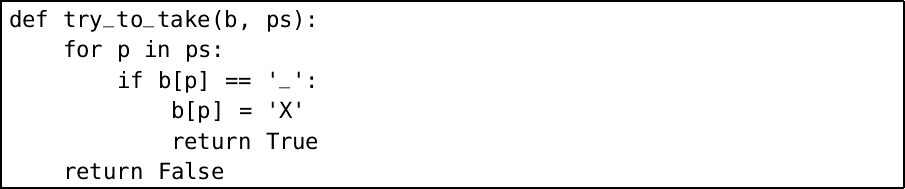Then, the tactic itself is simple:The ‘win’ and ‘block’ tactics are in fact similar to one another – if there is one space in a line, and two of our pieces we can win. If there is one space, and two of our opponents pieces, we must block. So we can write a single function which checks for this situation: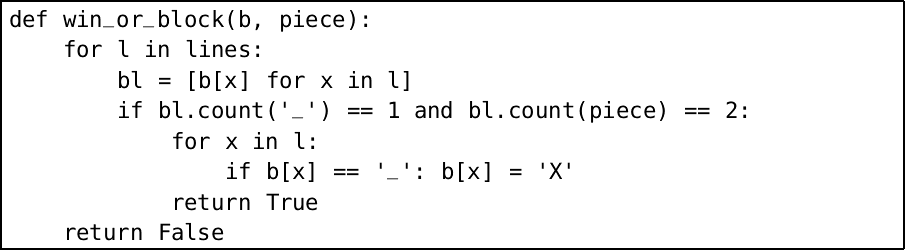Then the win and block tactics are simple: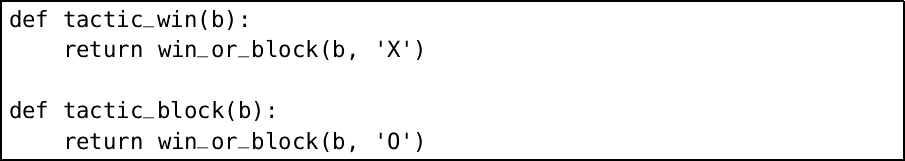We need a last-resort tactic which simply plays the first blank space:Now we can string the tactics together to build our `computer_move` function: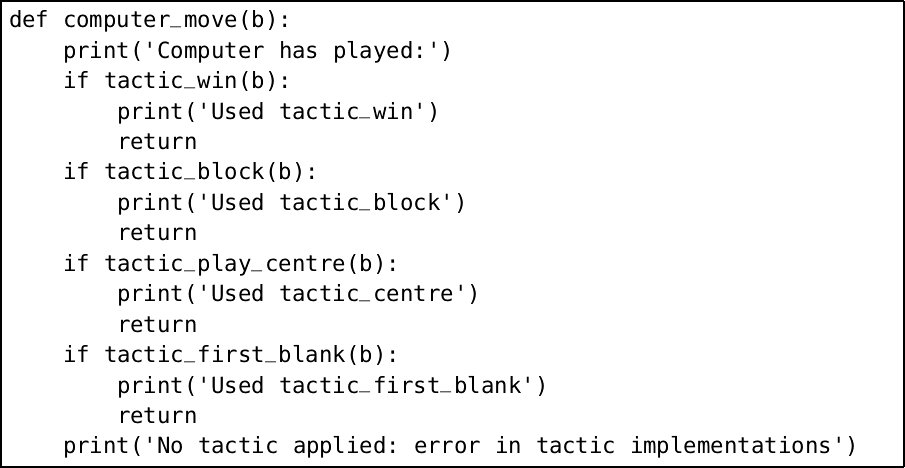Note that the `return` construct is used here to end the function early, not to return a value.

There is in fact a full, foolproof strategy for winning or forcing a draw. It consists, as we have suggested, of a list of rules to apply in turn, taking the first one which works. Here is the description, from “Flexible Strategy Use in Young Children’s Tic‐Tac‐Toe” by Kevin Crowley and Robert S. Siegler: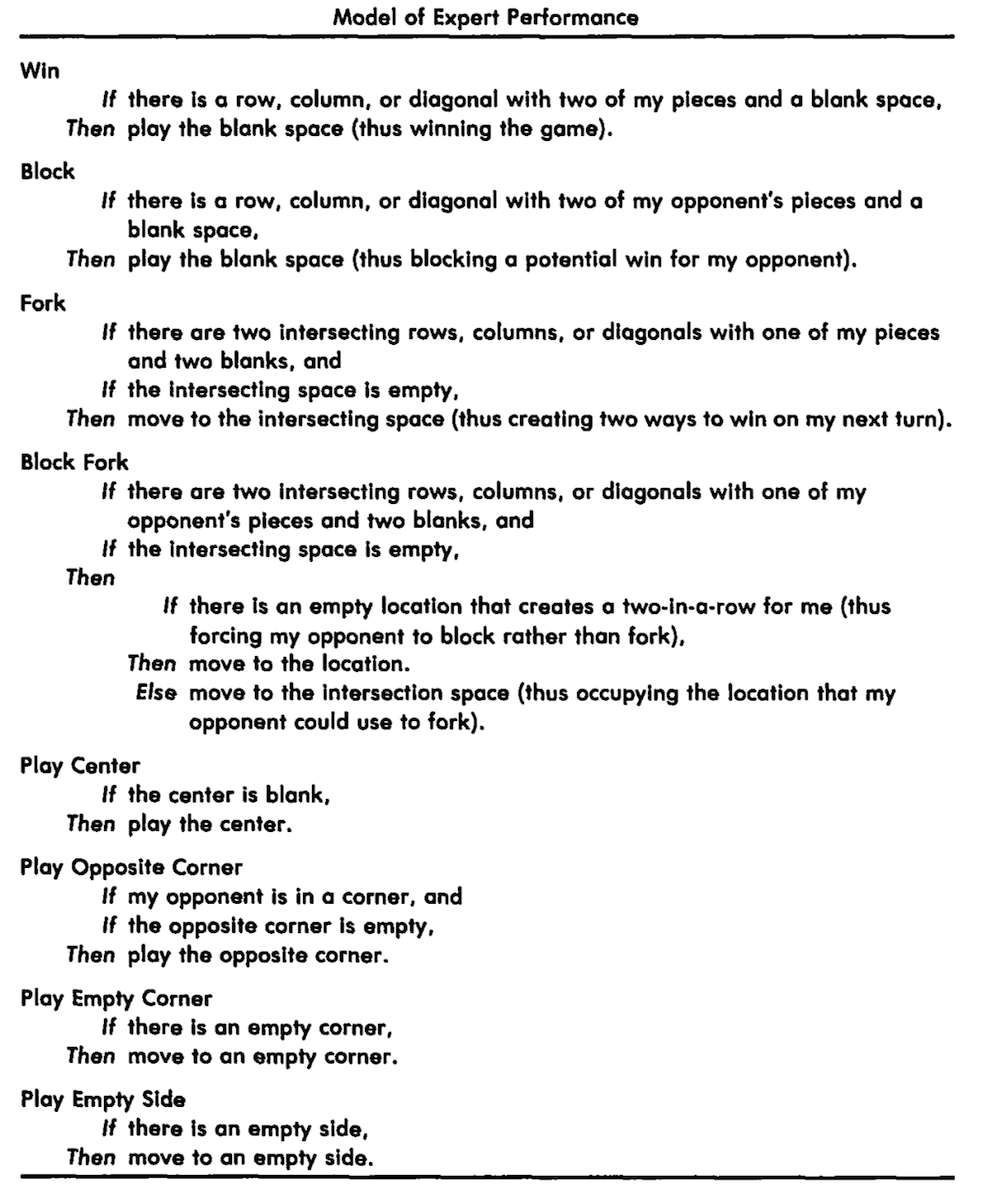You can see that the ‘Win’, ‘Block’ and ‘Center’ rules are the ones we have already implemented.

Question 6 Add the Empty Corner and Empty Side rules, allowing us to remove our First Blank rule. Add the Opposite Corner rule.

Question 7 Make a human-vs-computer game using your new player. Allow either player to make the first move, and make sure to print the intermediate and final state of the board.

Question 8 Implement the remaining two tactics, Fork and Block Fork, which are rather more complicated.

### All possible games

How many possible different games of Noughts and Crosses are there? How often does the player going first win, how often the playing going second, and how often is the game drawn? To answer these questions and more, we will construct what is known as a game tree. It is called a tree because it has a branching structure.

Let us consider the shape of the game tree when X begins. Clearly, at the top level, it will branch nine ways, since the starting player X can be placed in any square. Then eight ways, seven ways and so on. Once we reach the fifth level, some of the games have been won (since three pieces from player X may form a line), and so the number of branches may be reduced. The tree must end after nine levels, since the board must at least be full, even if no-one has won. Here is a fragment of the game tree: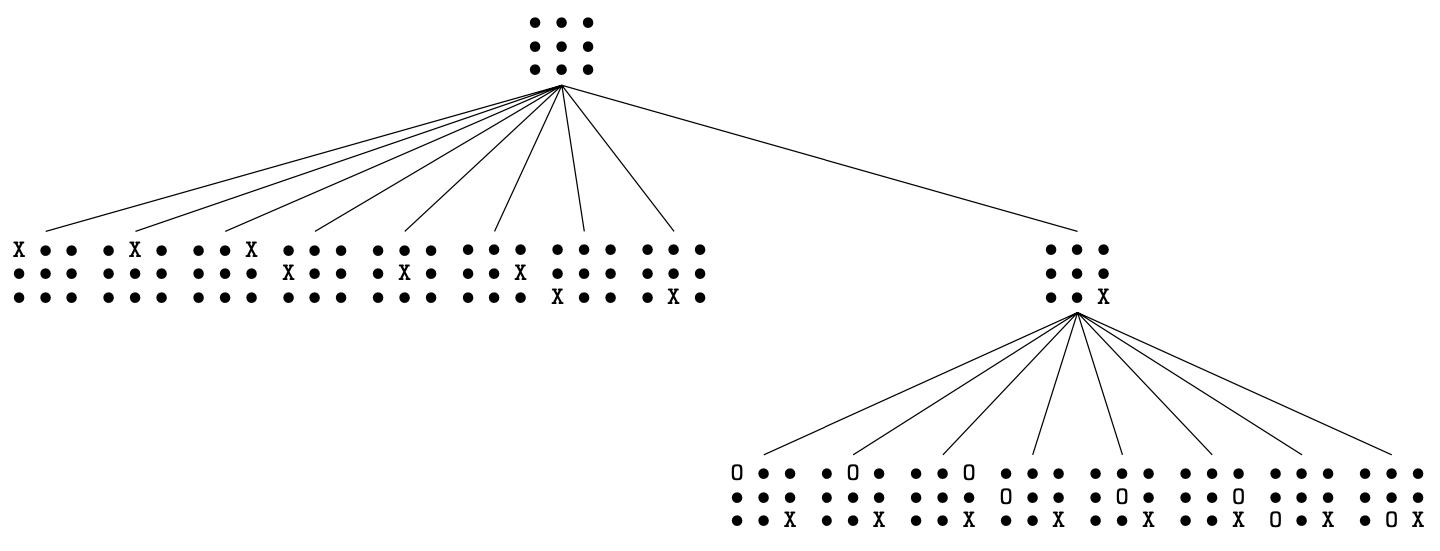How can we represent and construct the tree? We shall need to swap between players at each level to simulate turns, so we write a simple function for that: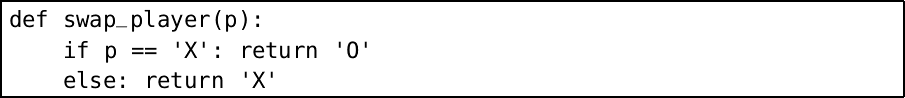Our data structure for the tree will be a pair `(b, bs)` where `b` is the current board, and `bs` a list of trees representing the possible successor trees after a move has taken place.

The function to build this tree is therefore recursive, just like the data structure. First it checks to see if the current board is won or drawn, in which case there are no successor boards. Otherwise, we produce a sub-tree for each possible move i.e. for each possible blank space in the board. We return the pairs of the board and the list of successor trees (produced by recursion), swapping the player each time.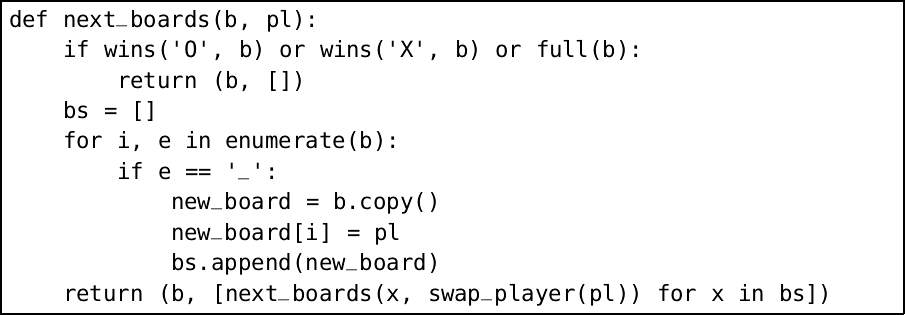We must be careful to copy the board using the list method `copy`, so that each list is mutated separately. Now we just need to set the process in motion, beginning with the empty board: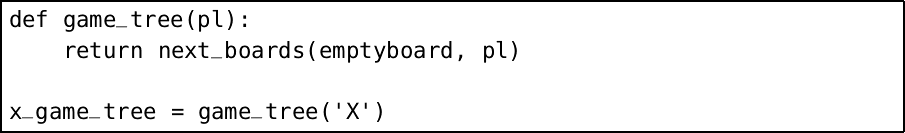This takes quite some time to run. The result is far too large to read on screen (you can print it just by writing `x_game_tree` and pressing Enter). We will therefore have to write some functions to interrogate the tree to derive statistics from it. For example, the following function tells us that X wins a game started by X 131184 times.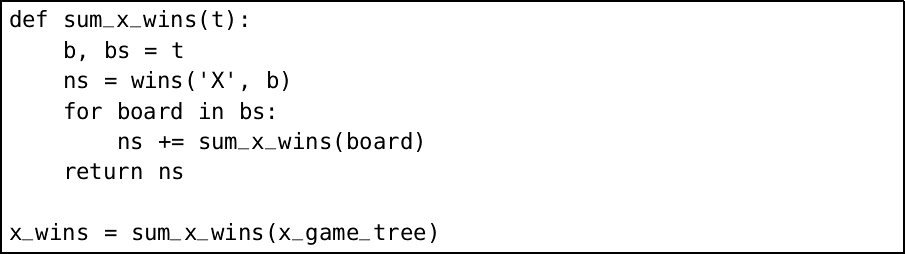Here, we are making use of the fact that `True` can be considered by Python to be the integer 1, and `False` the integer 0 when performing addition.

Question 9 In how many cases does O win? How many games end in a draw? How many possible different games are there?

Question 10 Write a function `sum_game_tree(f, t)` which takes a function and a tree, and gives the number of items in the tree for which the function is true. Use this to remove duplicate parts of your functions from question 9.

Question 11 Another use for a tree is in connection with our Morse code example from chapter 3. We can build a tree of our codes like this: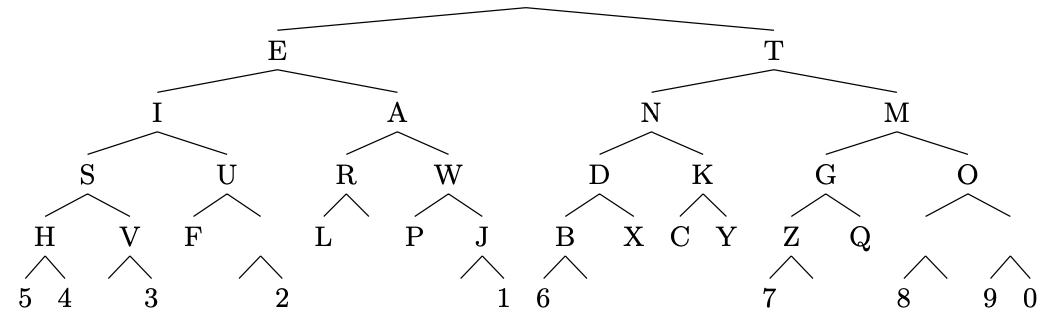Each line to the left is a dot, each to the right a dash. Encode this tree in Python, and use it to write a decoder for our Morse messages.